# Dimension Reduction III — Random Linear Projections and Locally Linear Embeddings

18 September 2019

$\newcommand{\X}{\mathbf{x}} \newcommand{\Y}{\mathbf{y}} \newcommand{\w}{\mathbf{w}} \newcommand{\V}{\mathbf{v}} \newcommand{\S}{\mathbf{s}} \newcommand{\Expect}{\mathbb{E}\left[ #1 \right]} \newcommand{\Var}{\mathrm{Var}\left[ #1 \right]} \newcommand{\SampleVar}{\widehat{\mathrm{Var}}\left[ #1 \right]} \newcommand{\Cov}{\mathrm{Cov}\left[ #1 \right]} \DeclareMathOperator{\tr}{tr} \DeclareMathOperator*{\argmin}{argmin}$

# Recap

• We have $$n$$ items represented as $$p$$-dimensional feature vectors; $$p$$ is too big
• PCA: Find the best $$q$$-dimensional linear approximation to the data
• $$\equiv$$ find the $$q$$-directions of maximum variance
• $$\equiv$$ find the top $$q$$ eigenvectors of the variance matrix
• Then project the $$p$$-dimensional feature vectors on to those eigenvectors
• Scores = lengths of the projections = new, $$q$$-dimensional features
• Factor analysis: Suppose the data are $$q$$-dimensional linear subspace plus noise
• Find the $$q$$-dimensional subspace which best preserves the correlations
• Backtrack to coordinates in the subspace (factor scores)
• Factor scores are the new, $$q$$-dimensional features
• Drawbacks:
• This is a lot of computational work!
• Who says linear subspaces are so great?

# PCA and distance preservation

• PCA: we project from $$\vec{x}_i$$ to $$\mathbf{u}\vec{x}_i$$
• $$\mathbf{u} =$$ projection operator for the principal components
• PCA: tries to make the projected points close to the original points, so $\frac{1}{n}\sum_{i=1}^{n}{\| \vec{x}_i - \mathbf{u}\vec{x}_i\|^2}$ is minimized
• This means distances between projected points track distances between original points
• Triangle inequality

# Distance-preserving projections

• Suppose we just want to preserve distances
• $$\mathbf{u}$$ would be distance preserving or an isometry if $\|\mathbf{u}\vec{x}_i - \mathbf{u}\vec{x}_j\|^2 = \|\vec{x}_i - \vec{x}_j\|^2$
• Exact distance-preservation is a lot to ask
• Unless $$p > n$$
• Approximate distance preservation: you pick an $$\epsilon > 0$$; $$\mathbf{u}$$ is an $$\epsilon$$-isometry when $(1-\epsilon) \|\vec{x}_i - \vec{x}_j\|^2 \leq \|\mathbf{u}\vec{x}_i - \mathbf{u}\vec{x}_j\|^2 \leq (1+\epsilon) \|\vec{x}_i - \vec{x}_j\|^2$
• A remarkable math fact: If $$q = O(\frac{\log{n}}{\epsilon^2})$$ then there is always an $$\epsilon$$-isometry

# The random projection trick

• Make $$Z_{ij} \sim \mathcal{N}(0,1)$$ independent standard Gaussians
• Set $\mathbf{U} = \frac{1}{\sqrt{q}}\left[\begin{array}{ccc} Z_{11} & Z_{12} & \ldots & Z_{1p}\\ Z_{21} & Z_{22} & \ldots & Z_{2p}\\ \vdots & \vdots & \ldots & \vdots\\ Z_{q1} & Z_{q2} & \ldots & Z_{qp}\end{array}\right]$
• $$\mathbf{U}\vec{x}_i$$ is like projecting on to $$d$$ random $$p$$-dimensional vectors
• What is the expected squared length of those vectors?
• Now you can check that for any vector $$\vec{x}$$, $\Expect{\|\mathbf{U}\vec{x}\|^2} = \|\vec{x}\|^2$
• Hint: $$\Expect{\|\mathbf{U}\vec{x}\|^2} = \Expect{\sum_{i=1}^{q}{\left(\frac{1}{\sqrt{q}}\sum_{j=1}^{p}{Z_{ij} x_j}\right)^2}}$$ and $$\|\vec{x}\|^2 = \sum_{j=1}^{p}{x_j^2}$$

# The random projection trick

• Expectation is linear and the $$Z_{ij}$$ are uncorrelated so $\Expect{\|\mathbf{U}\vec{x}\|^2} = \frac{1}{q}\sum_{i=1}^{q}{\sum_{j=1}^{p}{x_j^2}} = \|\vec{x}\|^2$

• Random projections are distance-preserving on average

# Random projections are nearly distance-preserving with high probability

• Fix your favorite $$\epsilon > 0$$ (approximation level) and $$\delta > 0$$ (error probability)
• Johnson-Lindenstrauss theorem: With probability at least $$1-\delta$$, $$\mathbf{U}$$ is an $$\epsilon$$-isometry for our $$n$$ data points if $q \geq \frac{8}{\epsilon^2}\log{\frac{n}{\sqrt{\delta}}}$
• E.g., for 95% confidence, need $$q \geq \frac{8}{\epsilon^2}\left(\log{(n)} + 1.498\ldots \right)$$
• For 95% confidence with $$n=205$$ and $$\epsilon=0.2$$, we need $$q \geq 1365$$
• If we had $$n=2\times 10^6$$, we’d need $$q \geq 3202$$
• The remarkable bits: $$p$$ doesn’t matter and neither does the data
• For the homework data, $$p=4.725\times 10^{5}$$
• You can also turn this around to see what $$\epsilon$$ you can achieve with a fixed budget of $$q$$
• With $$q = 100$$, $$n=205$$, $$\delta=0.05$$, we get $$\epsilon \approx 0.74$$ which isn’t great but is some signal
• Again, independent of $$p$$

# The problem with nonlinear structure

• Some nonlinear data (a nice spiral):
x = matrix(c(exp(-0.2 * (-(1:300)/10)) * cos(-(1:300)/10), exp(-0.2 * (-(1:300)/10)) *
sin(-(1:300)/10)), ncol = 2)
plot(x)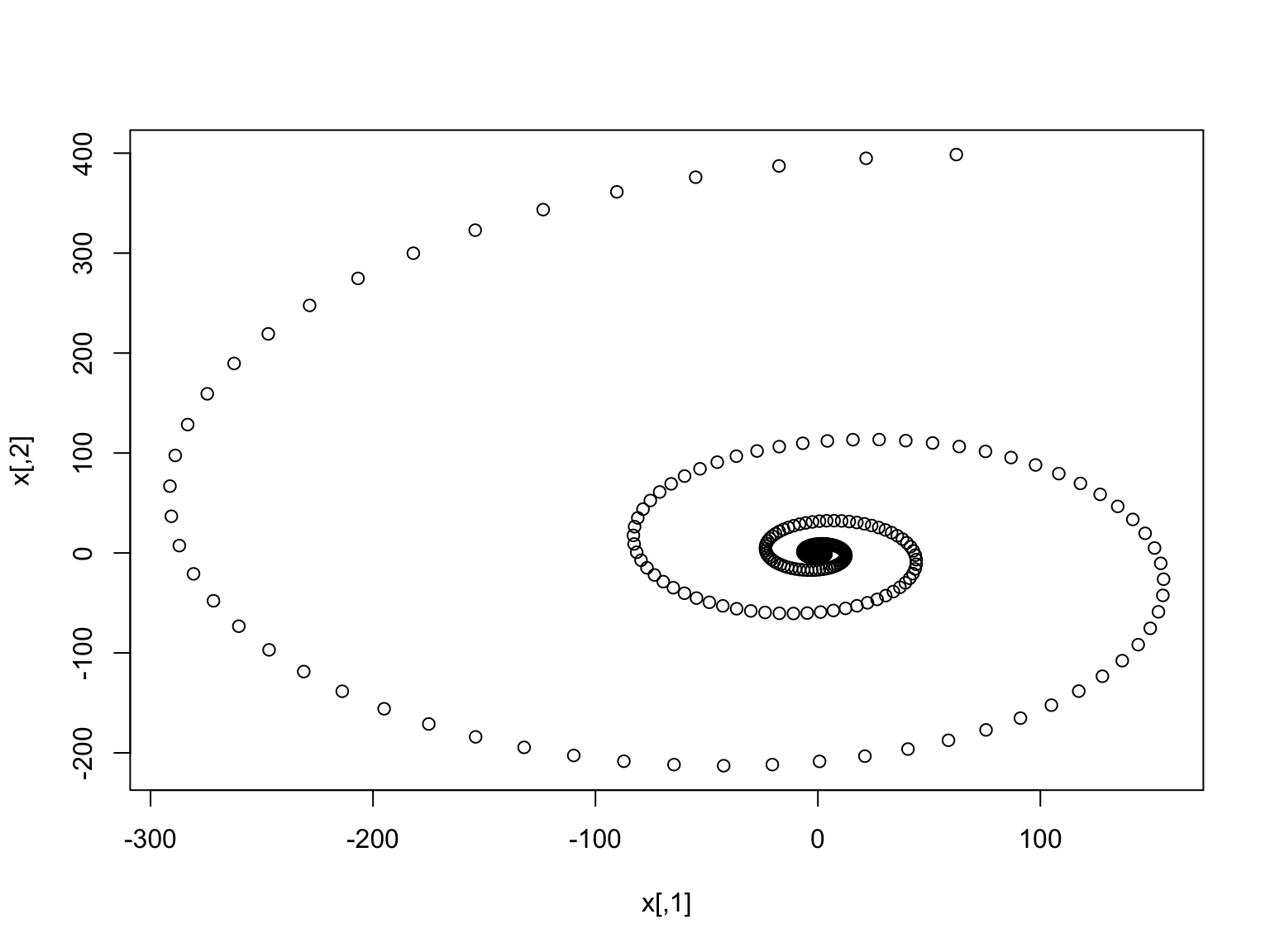# PCA just fails here

fit.all = prcomp(x)
approx.all = fit.all$x[, 1] %*% t(fit.all$rotation[, 1])
plot(x, xlab = expression(x), ylab = expression(x))
points(approx.all, pch = 4)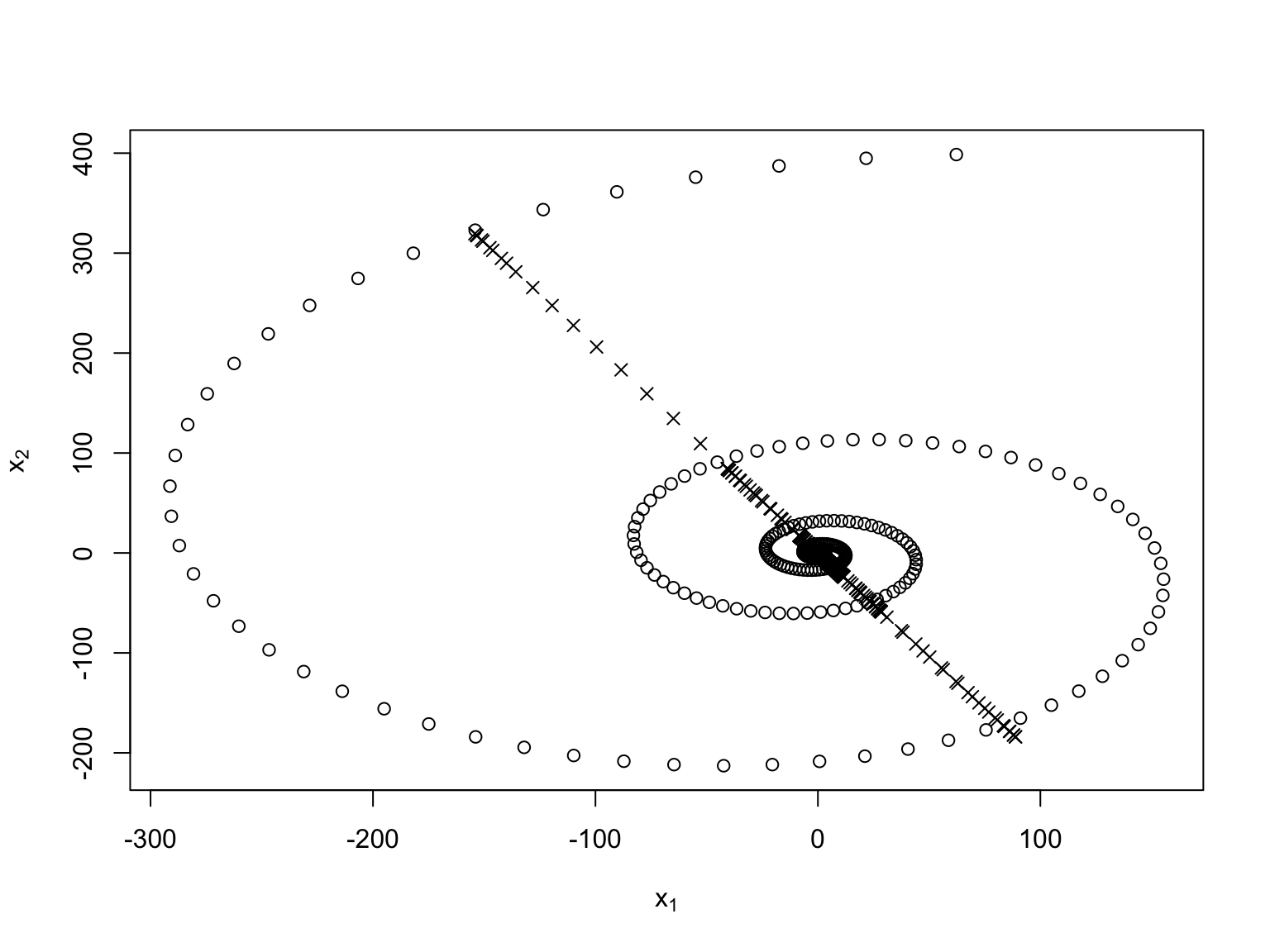# Manifold learning

• PCA and FA generally do badly when the data are curves (or curved surfaces)
• Geometry fact: locally, every smooth curved surface looks linear; a set we get by piecing these together is called a “manifold”
• Manifold learning = the data are on or near a manifold; find that manifold
• Factor analysis is a kind of linear manifold learning

# PCA doesn’t do too badly around any small part of the curve

fit = prcomp(x[270:280, ])
pca.approx = fit$x[, 1] %*% t(fit$rotation[, 1]) + colMeans(x[270:280, ])
plot(rbind(x[270:280, ], pca.approx), type = "n", xlab = expression(x), ylab = expression(x))
points(x[270:280, ])
points(pca.approx, pch = 4)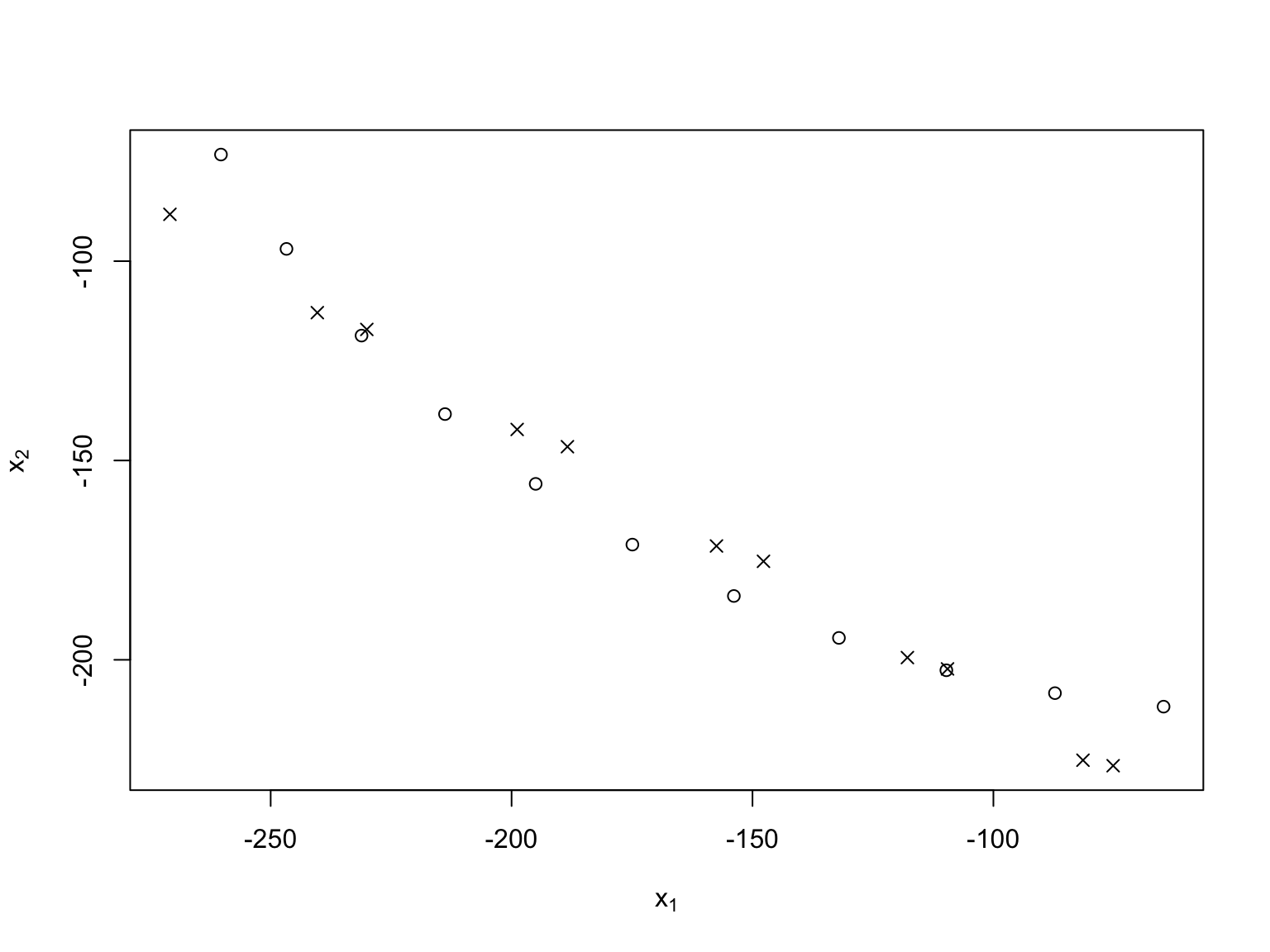# Local(ly) Linear Embedding

• Write each data point as a linear combination of near-by data points
• Look for lower-dimensional coordinates which fit those linear combinations

# LLE

• Inputs: $$\X$$ $$[n \times p]$$, $$q < p$$, $$k \geq q+1$$
• Output: $$\Y$$ $$[n \times q]$$
1. For each $$\vec{x}_i$$, find the $$k$$ nearest neighbors.
2. Find optimal weights for reconstructing $$\vec{x}_i$$ from its neighbors, i.e., minimize $\begin{equation} MSE(\mathbf{w}) \equiv \frac{1}{n}\sum_{i=1}^{n}{{\| \vec{x}_i - \sum_{j \neq i}{w_{ij} \vec{x}_j}\|}^2} \end{equation}$ with $$w_{ij} = 0$$ unless $$j$$ is a nearest neighbor of $$i$$, and $$\sum_{j}{w_{ij}} = 1$$
3. Find coordinates $$\Y$$ which minimize the reconstruction error, $\begin{equation} \Phi(\mathbf{Y}) \equiv \sum_{i=1}^{n}{{\|\vec{y}_i - \sum_{j\neq i}{w_{ij} \vec{y}_j}\|}^2} \end{equation}$ with constraints $$\sum_{i}{y_{ij}} = 0$$ and $$\Y^T \Y = \mathbf{I}$$ (centered, de-correlated)

# Finding neighbors in $$p$$-dimensional space

• We know how to do this

# Finding weights

$\min_{\w}{\frac{1}{n}\sum_{i=1}^{n}{{\| \vec{x}_i - \sum_{j \neq i}{w_{ij} \vec{x}_j}\|}^2}}$

• Can find weights separately for each data point $$\vec{x}_i$$
• i.e., independent computation of each row $$\w_{i\cdot}$$ so $\min_{\w_{i\cdot}}{\| \vec{x}_i - \sum_{j \neq i}{w_{ij} \vec{x}_j}\|^2}$
• If $$k \geq p+1$$, can always make the reconstruction error exactly 0
• Why $$k \geq p+1$$?
• If all points are in a $$q$$-dimensional linear subspace, reconstruction error could be made exactly 0 with only $$k \geq q+1$$ neighbors (why?)
• Non-zero minimum error tells us about local curvature / nonlinearity
• For big $$n$$, nearest neighbors should be close and we should be able to get close to 0

# Finding the weights

• A solve-a-linear-system problem, but a different one for each $$i$$
• In fact, another homework problem

# Finding the new coordinates

• We should be able to transfer the neighborhood structure from the old space to the new one
• Take the weights as fixed and ask for new, low-dimensional coordinates which match up with the weights: minimize $\Phi(\Y) = \sum_{i}{{\left\|\vec{y}_i - \sum_{j \neq i}{\vec{y}_j w_{ij}}\right\|}^2}$

• Yet another eigen-problem
• Yet another homework problem

# Implementation

# Local linear embedding of data vectors Inputs: n*p matrix of vectors,
# number of dimensions q to find (< p), number of nearest neighbors per
# vector, scalar regularization setting Calls: find.kNNs,
# reconstruction.weights, coords.from.weights Output: n*q matrix of new
# coordinates
lle <- function(x, q, k = q + 1, alpha = 0.01) {
stopifnot(q > 0, q < ncol(x), k > q, alpha > 0)  # sanity checks
kNNs = find.kNNs(x, k)  # should return an n*k matrix of indices
w = reconstruction.weights(x, kNNs, alpha)  # n*n weight  matrix
coords = coords.from.weights(w, q)  # n*q coordinate matrix
return(coords)
}
spiral.lle = lle(x, 1, 2)
plot(spiral.lle, ylab = "Coordinate on manifold")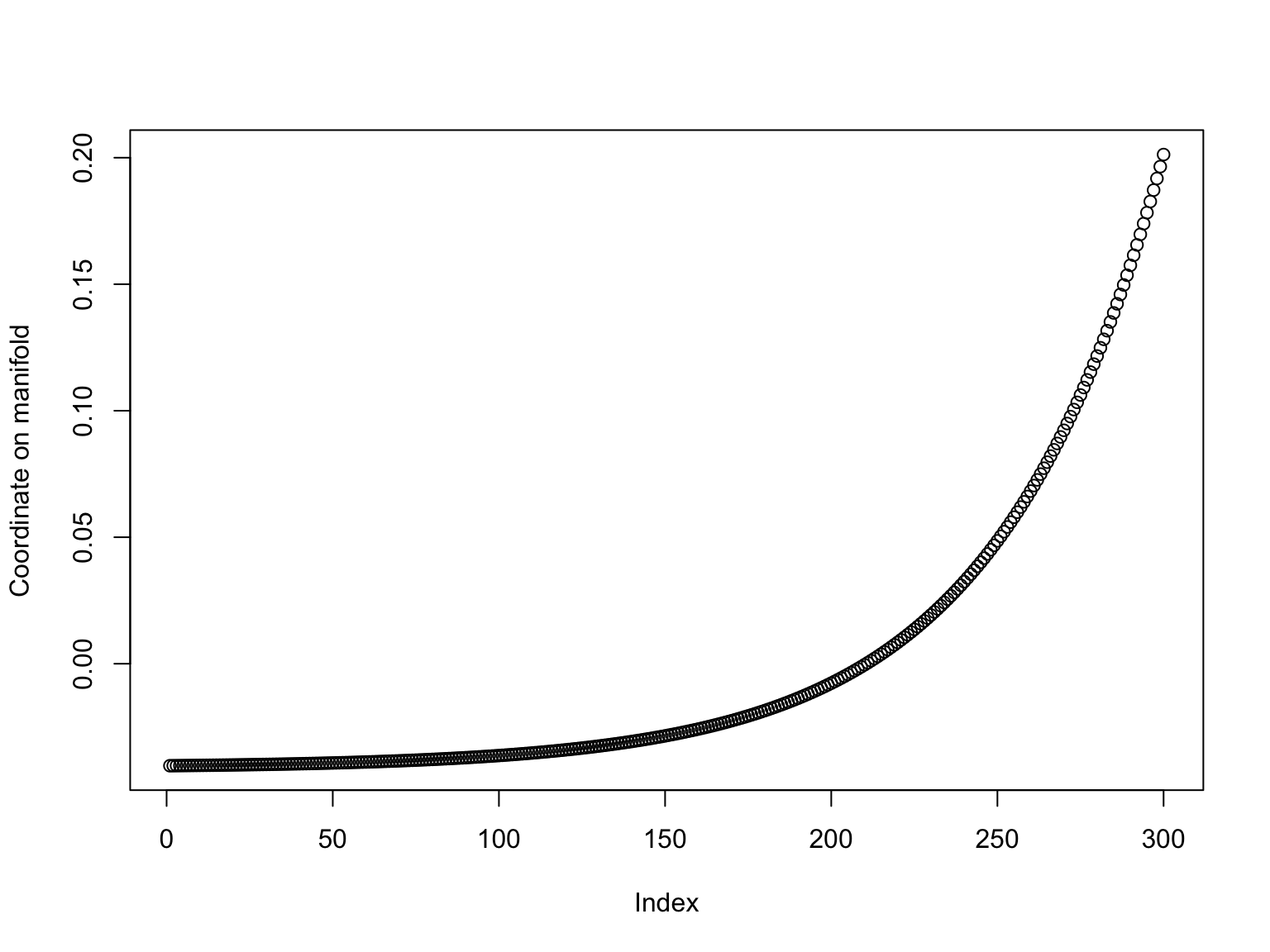all(diff(spiral.lle) > 0)
##  TRUE
plot(x, col = rainbow(300, end = 5/6)[cut(spiral.lle, 300, labels = FALSE)],
pch = 16)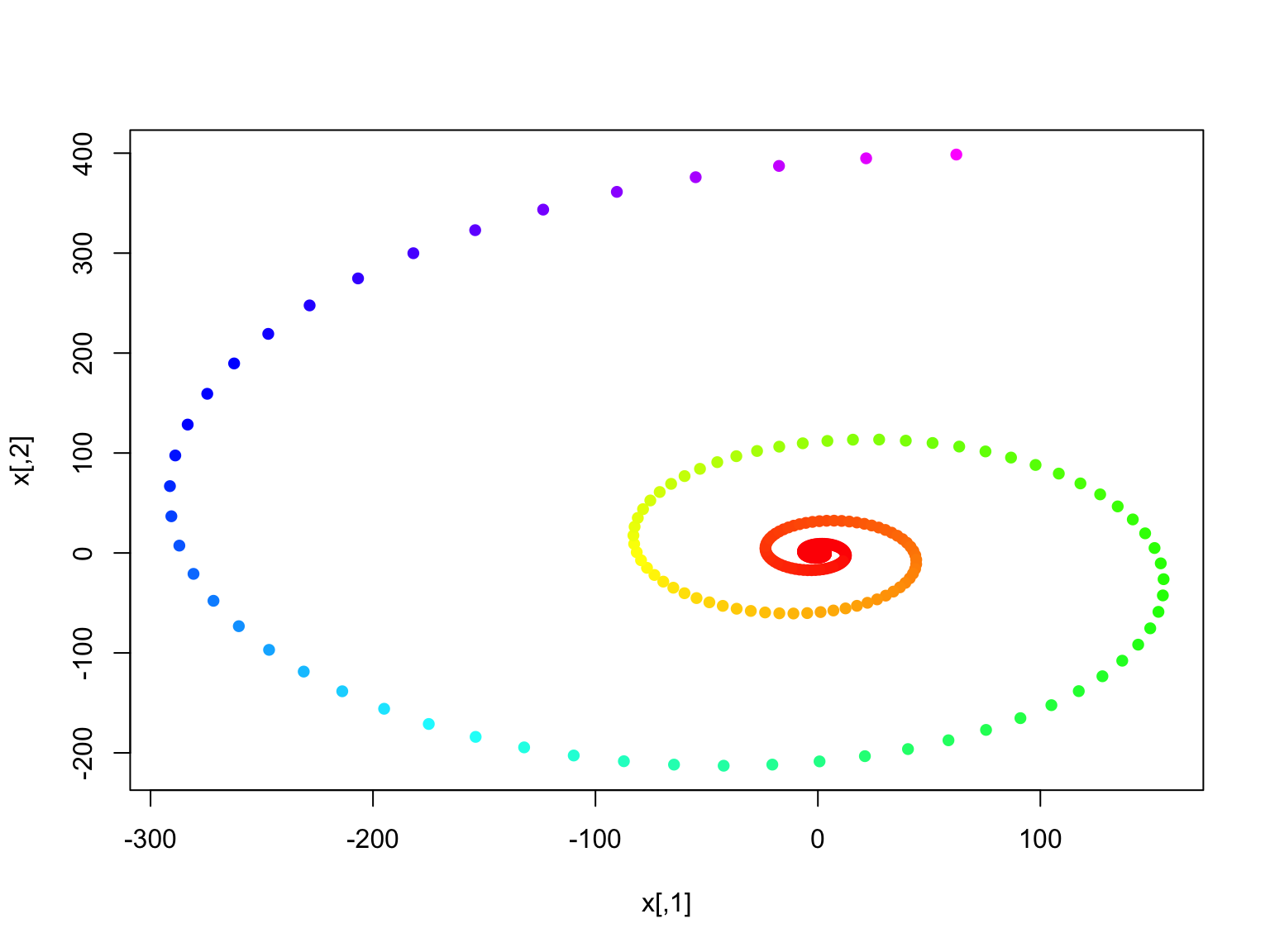# Afternotes

• There are many versions of the Johnson-Lindenstrauss Theorem on random projections, varying in what they assume about the random vectors and the exact proof technique
• The version given here, for Gaussian-entry random vectors, is taken from chapter 2 of Boucheron, Lugosi, and Massart (2013); because of the Gaussianity, it can be proved using just facts about $$\chi^2$$ distributions
• More general versions need more advanced probability theory, as in Boucheron, Lugosi, and Massart (2013)
• If you know what Fourier transforms are, and the Chernoff/Hoeffding bound on the probability of a random variable deviating from its expectation, then Suresh Venkatasubramanian gave a good explanation of the JL theorem in 2011
• (The result is often called the Johnson-Lindenstrauss “Lemma”, because it was just a lemma (subsidiary result) in their original paper)
• The JL Theorem is part of a broader family of results about using random samplings and projections to deal with high-dimensional linear algebra (e.g., linear regression for very big data sets); Mahoney (2011) is a good introduction
• Local linear embedding comes from Roweis and Saul (2000) and Saul and Roweis (2003)
• The source-code for these slides includes a complete (though not maximally efficient) implementation